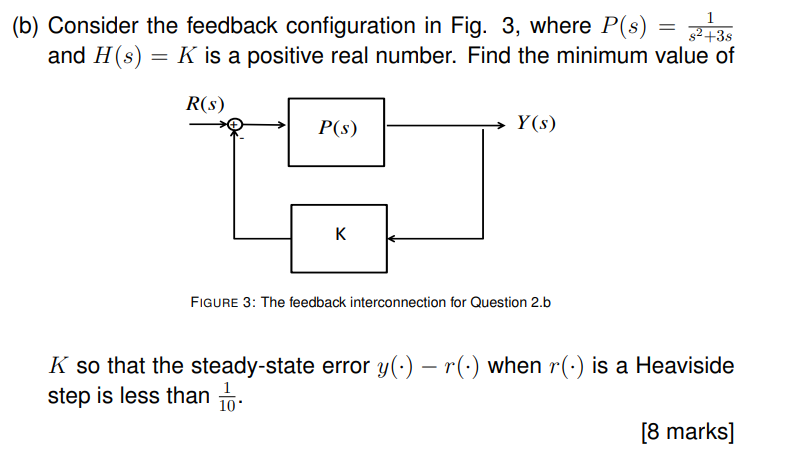Home / Expert Answers / Mechanical Engineering / b-consider-the-feedback-configuration-in-fig-3-where-p-s-frac-1-s-pa945

# (Solved): (b) Consider the feedback configuration in Fig. 3, where $$P(s)=\frac{1}{s^{ ...??????? (b) Consider the feedback configuration in Fig. 3, where \( P(s)=\frac{1}{s^{2}+3 s}$$ and $$H(s)=K$$ is a positive real number. Find the minimum value of FIGURE 3: The feedback interconnection for Question 2.b $$K$$ so that the steady-state error $$y(\cdot)-r(\cdot)$$ when $$r(\cdot)$$ is a Heaviside step is less than $$\frac{1}{10}$$.

We have an Answer from Expert